# 灰色预测模型

[TOC]

## 模型基本介绍

• 既含有已知信息又含有未知信息的系统：灰色系统
• 完全已知：白色系统
• 完全位置：黑色系统

### 灰色生成数列

• 常用的灰色系统生成方式有:
（1）累加生成
（2）累减生成
（3）均值生成
（4）级比生成

#### 累加生成

$X^{(0)}=\left(x^{(0)}(1), x^{(0)}(2), \ldots \ldots ., x^{(0)}(n)\right)$

$X^{(1)}=\left(x^{(1)}(1), x^{(1)}(2), \ldots \ldots ., x^{(1)}(n)\right)$

$x^{(1)}(k)=\sum_{i=0}^{k} x^{(0)}(i)=x^{(1)}(k-1)+x^{(0)}(k)$#### 累减生成

$X^{(1)}=\left(x^{(1)}(1), x^{(1)}(2), \ldots \ldots, x^{(1)}(n)\right)$

$X^{(0)}=\left(x^{(0)}(1), x^{(0)}(2), \ldots \ldots, x^{(0)}(n)\right)$

$x^{(0)}(k)=x^{(1)}(k)+x^{(1)}(k-1), \quad k=2,3, \ldots \ldots, n$

#### 邻值生成

$\mathrm{Z}^{(1)}$$\mathrm{X}^{(1)}$ 的紧邻均值 MEAN生成序列:

$Z^{(1)}=\left(z^{(1)}(2), z^{(1)}(3), \ldots \ldots ., z^{(1)}(n)\right)$

$z^{(1)}(k)=\alpha x^{(1)}(k)+(1-\alpha) x^{(1)}(k-1), \quad k=2,3, \ldots \ldots, n$

$\alpha$ 为生成系数，取0.5时，称生成的数列为均值生成数，也称等权邻值生成数。

### GM（1，1）模型

GM （1,1） 模型是灰色系统理论中应用最广泛的一种灰色动态预测模型，该模型由一个单变量的一阶微分方程构成。 它主要用于复杂系统某一主导因素特征值的拟合和预测，以揭示主导因素变化规律和未来发展变化态势。

#### 灰微分方程模型

$d(k)=x^{(0)}(k)=x^{(1)}(k)-x^{(1)}(k-1)$

$x^{(0)}(k)+a z^{(1)}(k)=b$

$d(k)+a z^{(1)}(k)=b$

$x^{(0)} 、 z^{(1)}(k)$ 为已知量, $a 、b$ 待求解， 故将 $k=2,3, \ldots, \mathrm{n}$ 待入， 可得到矩阵方程

$\left\{\begin{array}{l} x^{(0)}(2)+a z^{(1)}(2)=b, \\ x^{(0)}(3)+a z^{(1)}(3)=b \\ \ldots \ldots, \\ x^{(0)}(n)+a z^{(1)}(n)=b \end{array}\right.$

$\left\{\begin{array}{l} x^{(0)}(2)=-a z^{(1)}(2)+b, \\ x^{(0)}(3)=-a z^{(1)}(3)+b, \\ \ldots \ldots, \\ x^{(0)}(n)=-a z^{(1)}(n)+b \end{array}\right.$

$\begin{gathered} u=\left[\begin{array}{l} a \\ b \end{array}\right] \\ Y=\left[\begin{array}{c} x^{(0)}(2) \\ x^{(0)}(3) \\ \ldots \ldots \\ x^{(0)}(n) \end{array}\right] \\ B=\left[\begin{array}{cc} -z^{(1)}(2) & 1 \\ -z^{(1)}(3) & 1 \\ \cdots \cdots & \\ -z^{(1)}(n) & 1 \end{array}\right] \end{gathered}$

#### 白化方程模型

$\frac{d x^{(1)}(t)}{d t}+a x^{(1)}(t)=b$

$x^{(1)}(t)=\left(x^{(0)}(1)-\frac{b}{a}\right) e^{-a(t-1)}+\frac{b}{a}$

• 灰微分方程模型与白化方程模型的区别是什么？

灰微分方程里的初始序列 $x^{(0)}(k)$ 是离散的，白化方程里的初始序列是连续的。

而在正确使用这个模型的过程中，我们通过建立灰微分方程模型来求解 a ，b 并通过白化方程模型来求解 $x^{(1)}(t)$

## 算法步骤

### 1. 数据的检验与处理

$\lambda(k)=\frac{x^{(0)}(k-1)}{x^{(0)}(k)}, k=2,3, \ldots, n$

$y^{(0)}(k)=x^{(0)}(k)+C, k=1,2, \ldots, n$

### 2. 建立模型，求出预测值

$x^{(0)}(k)+a z^{(1)}(k)=b$

$\frac{d x^{(1)}(t)}{d t}+a x^{(1)}(t)=b$

$\begin{gathered} x^{(1)}(t)=\left(x^{(0)}(1)-\frac{b}{a}\right) e^{-a(t-1)}+\frac{b}{a} \\ \hat{x}^{(1)}(k+1)=\left(x^{(0)}(1)-\frac{b}{a}\right) e^{-a k}+\frac{b}{a} \\ \hat{x}^{(0)}(k)=\hat{x}^{(1)}(k)+\hat{x}^{(1)}(k-1), \quad k=2,3, \ldots \ldots, n \end{gathered}$

### 3. 检验预测值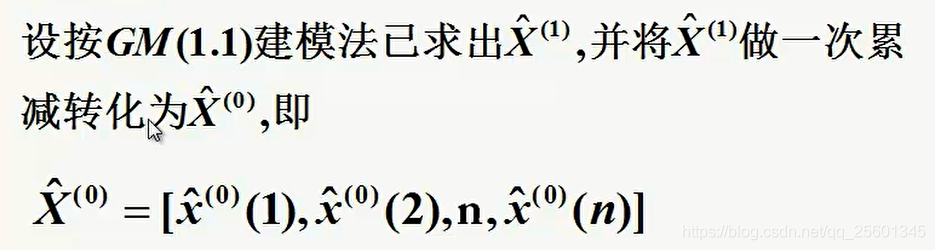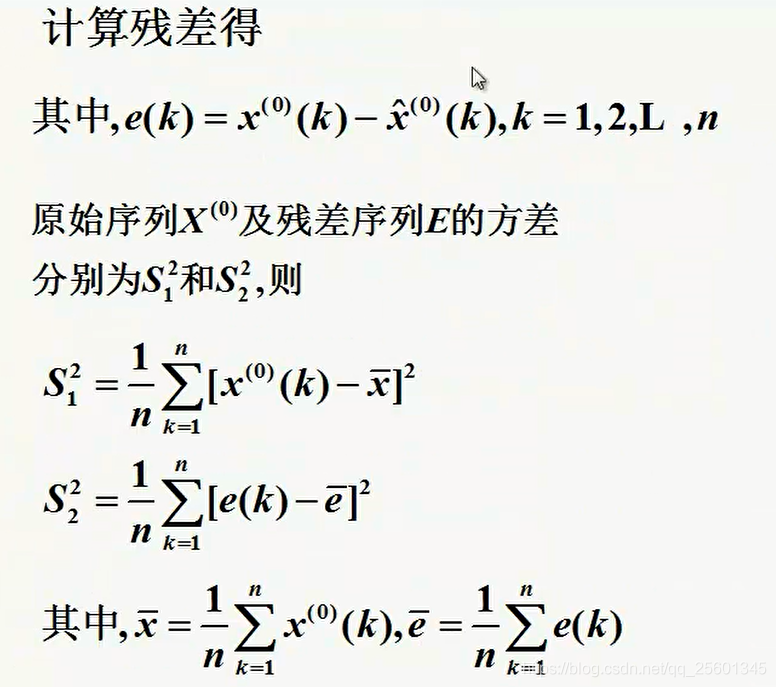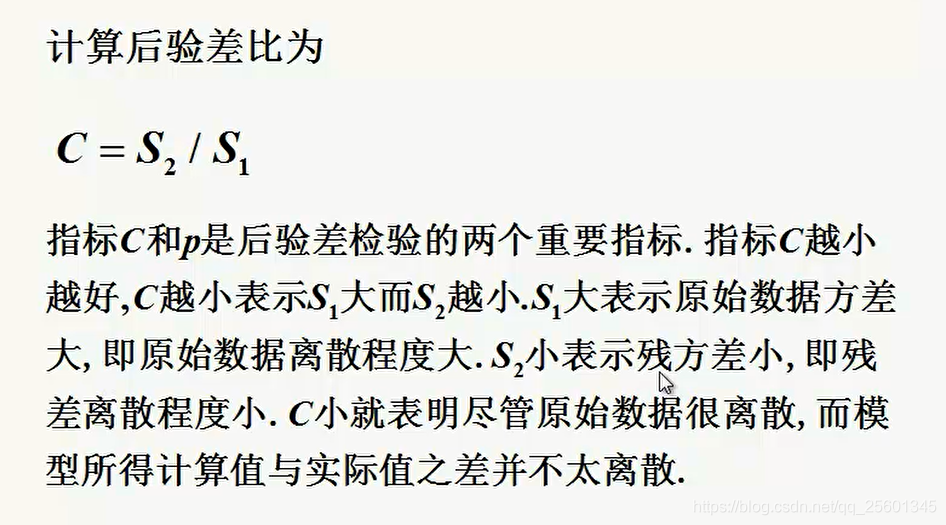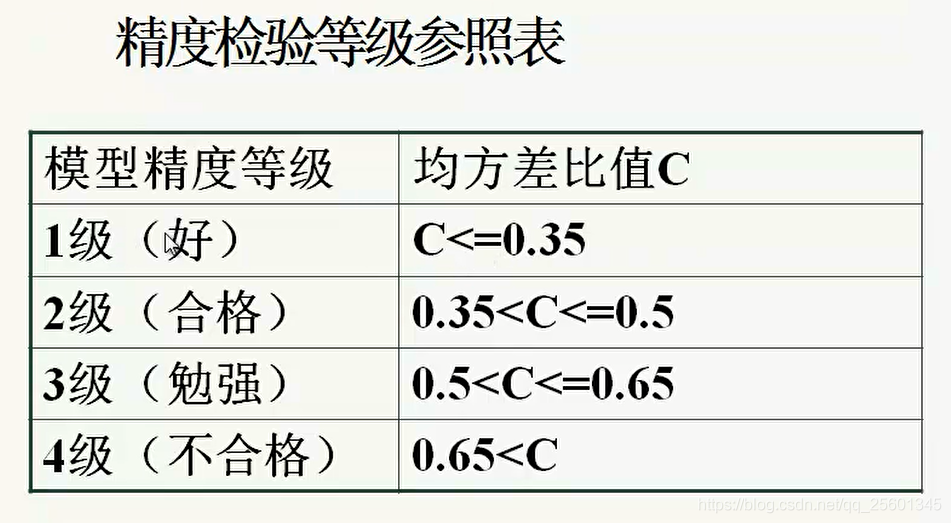• 计算相对残差

$\xi_{k}=\frac{x^{(0)}(k)-\hat{x}^{(0)}(k)}{x^{(0)}(k)}$

• 级比偏差值检验

$\rho(k)=1-\frac{1-0.5 a}{1+0.5 a} \lambda(k)$

## 应用实例（重要！！）

### 灰色预测计算实例

**例：**北方某城市 1986～1992 年道路交通噪声平均声级数据见表，请预测后几年的平均噪声：

1 1986 71.1 5 1990 71.4
2 1987 72.4 6 1991 72.0
3 1988 72.4 7 1992 71.6
4 1989 72.1

\begin{aligned} x^{(0)} &=\left(x^{(0)}(1), x^{(0)}(2), \cdots, x^{(0)}(7)\right) \\ &=(71.1,72.4,72.4,72.1,71.4,72.0,71.6) \end{aligned}

（1）求级比 $\lambda(k)$

$\lambda(k)=\frac{x^{(0)}(k-1)}{x^{(0)}(k)}$

\begin{aligned} \lambda &=(\lambda(2), \lambda(3), \cdots, \lambda(7)) \\ &=(0.982,1,1.0042,1.0098,0.9917,1.0056) \end{aligned}

（2）级比判断 由于所有的 $\lambda(k) \in[0.982,1.0098], k=2,3, \cdots, 7$, 故可以用 $x^{(0)}$ 作满意的GM（1，1）建模。

（1） 对原始数据 $x^{(0)}$ 作一次累加，即 $x^{(1)}=(71.1,143.5,215.9,288,359.4,431.4,503)$
（2） 构造数据矩阵 $B$ 及数据向量 $Y$

$B=\left[\begin{array}{cc} -\frac{1}{2}\left(x^{(1)}(1)+x^{(1)}(2)\right) & 1 \\ -\frac{1}{2}\left(x^{(1)}(2)+x^{(1)}(3)\right) & 1 \\ \vdots & \vdots \\ -\frac{1}{2}\left(x^{(1)}(6)+x^{(1)}(7)\right) & 1 \end{array}\right], \quad Y=\left[\begin{array}{c} x^{(0)}(2) \\ x^{(0)}(3) \\ \vdots \\ x^{(0)}(7) \end{array}\right]$

（3）计算 $\hat{u}$

$\hat{u}=(a, b)^{T}=\left(B^{T} B\right)^{-1} B^{T} Y=\left(\begin{array}{l} 0.0023 \\ 72.6573 \end{array}\right)$

（4）建立模型

$\frac{d x^{(1)}}{d t}+0.0023 x^{(1)}=72.6573$

$x^{(1)}(k+1)=\left(x^{(0)}(1)-\frac{b}{a}\right) e^{-a k}+\frac{b}{a}=-30929 e^{-0.0023 k}+31000$

（5） 求生成数列预测值 $\hat{x}^{(1)}(k+1)$ 及模型还原值 $\hat{x}^{(0)}(k+1)$ :

$k=1,2,3,4,5,6$, 由上面的时间响应函数可算得 $\hat{x}^{(1)}$, 其中取

\begin{aligned} &\hat{x}^{(1)}(1)=\hat{x}^{(0)}(1)=x^{(0)}(1)=71.1\\ &\text { 由 } \hat{x}^{(0)}(k)=\hat{x}^{(1)}(k)-\hat{x}^{(1)}(k-1), \text { 取 } k=2,3,4, \cdots, 7, \text { 得 }\\ &\hat{x}^{(0)}=\left(\hat{x}^{(0)}(1), \hat{x}^{(0)}(2), \cdots, \hat{x}^{(0)}(7)\right)=(71.1,72.4,72.2,72.1,71.9,71.7,71.6) \end{aligned}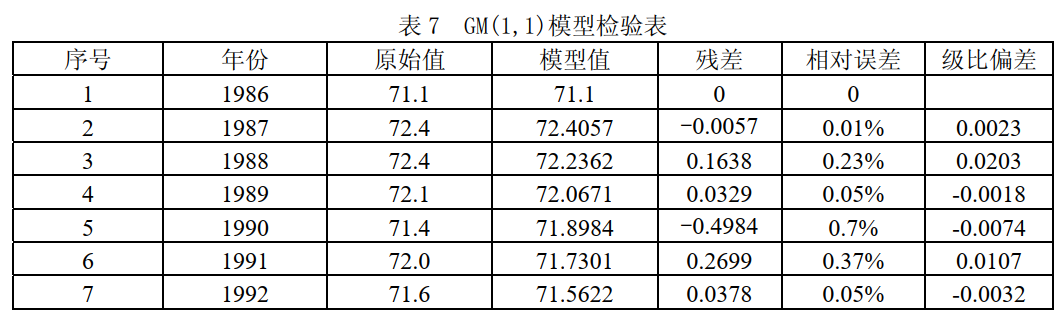### 灾变预测

$x_{\zeta}^{0}=(3,8,5)$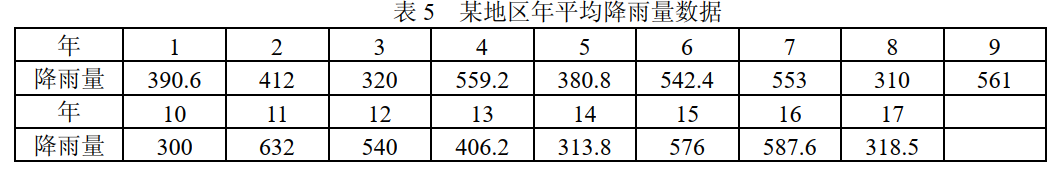$\begin{gathered} x^{(0)}=(390.6,412,320,559.2,380.8,542.4,553,310,561,300,632, \\ 540,406.2,313.8,576,587.6,318.5) \end{gathered}$

$x_{\zeta}^{0}=(320,310,300,313.8,318.5)$

$t=(3,8,10,14,17)$

$t^{(1)}=(3,11,21,35,52)$

\begin{aligned} &\hat{u}=(a, b)^{T}=(-0.2536,6.2585) \\ &\hat{t}^{(1)}(k+1)=27.6774 e^{0.2536 k}-24.6774 \end{aligned}

$t^{(0)}(6)=22.034, \quad t^{(0)}(7)=28.3946$

• 所需建模信息少

• 运算方便

• 建模精度高

## 参考博文及文献

【数学建模】灰色预测模型GM(1，1)附例题分析(MATLAB实现)

【数学建模】灰色预测模型（预测）

【数学建模】灰色预测模型

《数学建模算法与应用》------司守奎